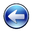# 程序控

IPPP (Institute of Penniless Peasent-Programmer) Fellow

 博客园 :: 首页 :: 博问 :: 闪存 :: 新随笔 :: 联系 :: :: 管理 ::（敬告：当您的浏览器以非默认字体浏览本文时，段落格式可能会出现偏差）

## 一、综述

cout是STL库提供的一个iostream实例，拥有ios_base基类的全部函数和成员数据。进行格式化操作可以直接利用setf/unsetf函数和flags函数。cout维护一个当前的格式状态，setf/unsetf函数是在当前的格式状态上追加或删除指定的格式，而flags则是将当前格式状态全部替换为指定的格式。cout为这个函数提供了如下参数（可选格式）：

• ios::dec  以10进制表示整数
• ios::hex  以16进制表示整数
• ios::oct  以8进制表示整数
• ios::showbase  为整数添加一个表示其进制的前缀
• ios::internal  在符号位和数值的中间插入需要数量的填充字符以使串两端对齐
• ios::left  在串的末尾插入填充字符以使串居左对齐
• ios::right  在串的前面插入填充字符以使串居右对齐
• ios::boolalpha  将bool类型的值以true或flase表示，而不是1或0
• ios::fixed  将符点数按照普通定点格式处理（非科学计数法）
• ios::scientific  将符点数按照科学计数法处理（带指数域）
• ios::showpoint  在浮点数表示的小数中强制插入小数点（默认情况是浮点数表示的整数不显示小数点）
• ios::showpos  强制在正数前添加+号
• ios::skipws  忽略前导的空格（主要用于输入流，如cin）
• ios::unitbuf  在插入（每次输出）操作后清空缓存
• ios::uppercase  强制大写字母

cout.setf(ios::right | ios::hex); //设置16进制右对齐
cout.setf(ios::right, ios::adjustfield); //取消其它对齐，设置为右对齐

setf可接受一个或两个参数，一个参数的版本为设置指定的格式，两个参数的版本中，后一个参数指定了删除的格式。三个已定义的组合格式为：

• ios::basefield  进制的组合位
• ios::floatfield  浮点表示方式的组合位

#include <iomanip>
#include <iostream>

## 二、缩进

#include <iomanip>
#include <iostream>
using namespace std;
int main(void) {
cout.flags(ios::left); //左对齐
cout << setw(10) << -456.98 << "The End" << endl;
cout.flags(ios::internal); //两端对齐
cout << setw(10) << -456.98 << "The End" << endl;
cout.flags(ios::right); //右对齐
cout << setw(10) << -456.98 << "The End" << endl;
return 0;
}

#include <iomanip>
#include <iostream>
using namespace std;
int main(void) {
cout << left << setw(10) << -456.98 << "The End" << endl; //左对齐
cout << internal << setw(10) << -456.98 << "The End" << endl; //两端对齐
cout << right << setw(10) << -456.98 << "The End" << endl; //右对齐
return 0;
}

-456.98   The End
-   456.98The End
-456.98The End

#include <iomanip>
#include <iostream>
using namespace std;
int main(void) {
cout << setfill('0') << setw(10) << 45698 << endl;
return 0;
}

0000045698

## 三、整数

#include <iomanip>
#include <iostream>
using namespace std;
int main(void) {
cout.setf(ios::showpos | ios::uppercase);
cout << hex << setw(4) << 12 << setw(12) << -12 << endl;
cout << dec << setw(4) << 12 << setw(12) << -12 << endl;
cout << oct << setw(4) << 12 << setw(12) << -12 << endl;
cout.unsetf(ios::showpos | ios::uppercase);
cout << hex << setw(4) << 12 << setw(12) << -12 << endl;
cout << dec << setw(4) << 12 << setw(12) << -12 << endl;
cout << oct << setw(4) << 12 << setw(12) << -12 << endl;
return 0;
}

C    FFFFFFF4
+12         -12
14 37777777764
c    fffffff4
12         -12
14 37777777764

#include <iomanip>
#include <iostream>
using namespace std;
int main(void) {
cout << showbase << setw(4) << hex << 32 << setw(4) << oct << 32 << endl;
cout << noshowbase << setw(4) << hex << 32 << setw(4) << oct << 32 << endl;
return 0;
}



0x20 040
20  40

#include <iomanip>
#include <iostream>
using namespace std;
int main(void) {
cout.setf(ios::showbase);
cout << setw(4) << hex << 32 << setw(4) << oct << 32 << endl;
cout.unsetf(ios::showbase);
cout << setw(4) << hex << 32 << setw(4) << oct << 32 << endl;
return 0;
}


## 四、小数

#include <iomanip>
#include <iostream>
using namespace std;
int main(void) {
cout.setf(ios::fixed);
cout << setprecision(0) << 12.05 << endl;
cout << setprecision(1) << 12.05 << endl;
cout << setprecision(2) << 12.05 << endl;
cout << setprecision(3) << 12.05 << endl;
cout.setf(ios::scientific, ios::floatfield);
cout << setprecision(0) << 12.05 << endl;
cout << setprecision(1) << 12.05 << endl;
cout << setprecision(2) << 12.05 << endl;
cout << setprecision(3) << 12.05 << endl;
return 0;
}


12
12.1
12.05
12.050
1.205000e+001
1.2e+001
1.21e+001
1.205e+001

#include <iomanip>
#include <iostream>
using namespace std;
int main(void) {
cout << fixed << setprecision(1) << 2.05 << endl;
cout << fixed << setprecision(1) << 2.05 + 1e-8 << endl;
return 0;
}


2.0
2.1

## 四、字符串

#include <iomanip>
#include <iostream>
#include <string>
using namespace std;
int main(void) {
string str1, str2;
getline(cin, str1);
cin >> str2;
cout << str1 << endl << str2 << endl;
return 0;
}


abc
abc

abc
abc

## 五、缓冲区

flush和endl都会将当前缓冲区中的内容立即写入到屏幕上，而unitbuf/nounitbuf可以禁止或启用缓冲区。示例代码如下：

#include <iomanip>
#include <iostream>
using namespace std;
int main(void) {
cout << 123 << flush << 456 << endl;
cout << unitbuf << 123 << nounitbuf << 456 << endl;
return 0;
}


123456
123456

## 六、综合使用

#include <iomanip>
#include <iostream>
#include <string>
using namespace std;
struct COMMODITY { string Name; int Id; int Cnt; double Price; };
int main(void) {
COMMODITY cmd[] = {
{"Fruit", 0x101, 50, 5.268},
{"Juice", 0x102, 20, 8.729},
{"Meat", 0x104, 30, 10.133},
};
cout << left << setw(8) << "NAME" << right << setw(8) << "ID";
cout << right << setw(8) << "COUNT" << right << setw(8) << "PRICE" << endl;
for (int i = 0; i < sizeof(cmd) / sizeof(cmd); ++i) {
cout << left << setw(8) << cmd[i].Name;
cout << right << hex << showbase << setw(8) << cmd[i].Id;
cout << dec << noshowbase << setw(8) << cmd[i].Cnt;
cout << fixed << setw(8) << setprecision(2) << cmd[i].Price << endl;
}
return 0;
}


NAME          ID   COUNT   PRICE
Fruit      0x101      50    5.27
Juice      0x102      20    8.73
Meat       0x104      30   10.13返回我的文章目录作者：王雨濛；新浪微博：@吉祥村码农；来源：《程序控》博客 -- http://www.cnblogs.com/devymex/ 此文章版权归作者所有（有特别声明的除外)，转载必须注明作者及来源。您不能用于商业目的也不能修改原文内容。

posted on 2010-09-06 12:08  Devymex  阅读(56290)  评论(7编辑  收藏  举报